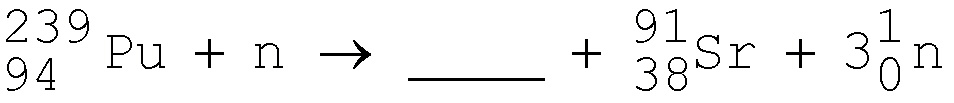CHEM 1200

Practice Test 5

Note: These questions are representative of the types of questions you can expect on the test. Use tables of reduction potentials from the textbook. The actual test will be longer, typically between 20-40 questions. You will need to bring a SCANTRON answer sheet to each test.

1. The electron configuration of a Fe2+ ion is:

a. [Ar]4s24d4
b. [Ar]4s23d6
c. [Ar]3d3
d. [Ar]3d5
e. [Ar]3d6

2. How many 3d electrons does a Mn2+ ion have?

a. 1
b. 2
c. 3
d. 4
e. 5

3.Which of the following ligands produces the weakest crystal field?

a. Br-
b. Cl-
c. H2O
d. NH3
e. CN-

4. In the complex ion [Fe(CN)6]4-, the oxidation number of Fe is:

a. +1
b. +2
c. +3
d. - 4
e. +6

5. Which one of the following is a bidentate ligand?

a. CN-
b. NH3
c. CO
d. H2NCH2CH2NH2 (ethylenediamine)
e. EDTA

6. The best name for [Ru(NH3)2(en)](NO3)2 is:

a. (ethylenediamine)diammineruthenium(II) nitrate
b. diamminebis(ethylenediamine)ruthenium(III) nitrate
c. diammine(ethylenediamine)ruthenium(II) nitrate
d. diammine(ethylenediamine)nitratoruthenium(III)
e .bis(ethylene)diaminenitratoruthenate(II)

7. In the complex ion ML6n+, Mn+ has seven d electrons and L is a weak field ligand. According to crystal field theory the magnetic properties of the complex ion correspond to how many unpaired electrons?

a. 0
b. 1
c. 2
d. 3
e. 5

8. The complex ion [Cr(CN)6]4- has magnetic properties that correspond to how many unpaired electrons?

a. 0
b. 1
c. 2
d. 3
e. 4

9. The ion [Co(NH3)6]2+ is octahedral and high spin. This complex is:

a. paramagnetic, with 1 unpaired electron.
b. paramagnetic, with 3 unpaired electrons.
c. paramagnetic, with 4 unpaired electrons.
d. paramagnetic, with 5 unpaired electrons.
e. diamagnetic

10. What geometry does [CoF6]3- exhibit?

a. tetrahedral
b. octahedral
c. square planar
d. trigonal bipyramidal

11. Which formula corresponds to tetramminecopper(II) sulfate?

a. [Cu(NH3)]SO4
b. [Cu(NH3)4]SO4
c. [Cu(NH3)5]SO4
d. [Cu2(NH2)2]SO4
e. [Cu2(NH3)4]SO4

12. Beta particles are identical to:

a. protons
b. helium atoms
c. hydrogen atoms
d. helium nuclei
e electrons

13. A radioisotope decays to give an alpha particle and Pb-208. What was the original element?

a. Se
b. Bi
c. Po
d. Hg
e. Rn

14. What is the missing symbol in this plutonium fission reaction?a. barium-148
b. a beta particle
c. xenon-143
d. strontium-91
e. barium-146

15. Calculate the total nuclear binding energy in joules for Mg-25 (atomic mass = 24.985839 amu).
Given: mass of proton = 1.007825 amu; mass of neutron = 1.008665 amu; 1 kg = 1.66 x 10-27 amu and c = 3.00 x 108 m/sec.

a. 0.22076 J
b. 3.30 x 10-11 J
c. 1.32 x 10-12 J
d. 0.999 J
e. none of the above

16. Charcoal found under a stone at Stonehenge, England, has a carbon–14 activity that is 0.60 that of new wood. How old is the charcoal? The half-life of carbon–14 is 5,730 years.

a. less than 5,730 yr
b. between 5,730 and 11,460 yr
c. between 11,460 and 17,190 yr
d. more than 17,190 yr

***************************